• labml.ai Deep Learning Paper Implementaion This is a collection of simple PyTorch implementations of neural networks and related algorithms. These implementations are documented with explanations, ...
• DQN（Deep Q-Learning Network）可谓是深度强化学习（Deep Reinforcement Learning，DRL）的开山之作，是将深度学习与强化学习结合起来从而实现从感知（Perception）到动作（ Action ）的端对端（End-to-end）学习的...

DQN（Deep Q-Learning Network）可谓是深度强化学习（Deep Reinforcement Learning，DRL）的开山之作，是将深度学习与强化学习结合起来从而实现从感知（Perception）到动作（ Action ）的端对端（End-to-end）学习的一种全新的算法。由DeepMind在NIPS 2013上发表1，后又在Nature 2015上提出改进版本2。

DQN（Deep Q-Learning Network）创新点：

基于Q-Learning构造Loss Function（不算很新，过往使用线性和非线性函数拟合Q-Table时就是这样做）。
通过experience replay（经验池）解决相关性及非静态分布问题；
使用TargetNet解决稳定性问题。

DQN（Deep Q-Learning Network）优点：

算法通用性，可玩不同游戏；
End-to-End 训练方式；
可生产大量样本供监督学习。

DQN（Deep Q-Learning Network）缺点：

无法应用于连续动作控制；
只能处理只需短时记忆问题，无法处理需长时记忆问题（后续研究提出了使用LSTM等改进方法）；
CNN不一定收敛，需精良调参。

一、Deep “Reinforcement Learning”

在普通的Q-learning中，当状态和动作空间是离散且维数不高时可使用Q-Table储存每个状态动作对的Q值，而当状态和动作空间是高维连续时，使用Q-Table不现实。通常做法是把Q-Table的更新问题变成一个函数拟合问题，相近的状态得到相近的输出动作。如下式，通过更新参数 θ 使Q函数逼近最优Q值
$Q(s,a;θ)≈Q'(s,a)$
而深度神经网络可以自动提取复杂特征，因此，面对高维且连续的状态使用深度神经网络最合适不过了。
DRL(Deep Reinforcement Learning)是将深度学习（DL）与强化学习（RL）结合，直接从高维原始数据学习控制策略。而DQN是DRL的其中一种算法，它要做的就是将卷积神经网络（CNN）和Q-Learning结合起来。

DQN中的CNN的输入是原始图像数据（作为状态State），
DQN中的Q-Learning输出则是每个动作Action对应的价值评估Value Function（Q值）。

二、DL(Deep Learning)与RL(Reinforcement Learning)结合的问题

DL需要大量带标签的样本进行监督学习；RL只有reward返回值，而且伴随着噪声，延迟（过了几十毫秒才返回），稀疏（很多State的reward是0）等问题；
DL的样本独立；RL前后state状态相关；
DL目标分布固定；RL的分布一直变化，比如你玩一个游戏，一个关卡和下一个关卡的状态分布是不同的，所以训练好了前一个关卡，下一个关卡又要重新训练；
过往的研究表明，使用非线性网络表示值函数时出现不稳定等问题。

三、DQN(Deep Q-Learning Network)解决DL与RL结合的问题的方法

通过Q-Learning使用reward来构造标签（对应问题1）
通过experience replay（经验池）的方法来解决相关性及非静态分布问题（对应问题2、3）
使用一个CNN（MainNet）产生当前Q值，使用另外一个CNN（Target）产生Target Q值（对应问题4）

1、构造标签

前面提到DQN中的CNN作用是对在高维且连续状态下的Q-Table做函数拟合，而对于函数优化问题，监督学习的一般方法是先确定Loss Function，然后求梯度，使用随机梯度下降等方法更新参数。DQN则基于Q-Learning来确定Loss Function。
Q-Learning的更新公式：
$Q^∗(s,a)=Q(s,a)+α[r+γ\max_{a'}Q(s',a')−Q(s,a)]$
DQN的Loss Function为：
$L(θ)=E[(TargetQ−Q(s,a;θ))^2]$
其中 θ 是网络参数
目标为
$TargetQ=r+γ\max_{a′}Q(s',a';θ)$
显然Loss Function是基于Q-Learning更新公式的第二项确定的，两个公式意义相同，都是使当前的Q值逼近Target Q值。
接下来，求 $L(θ)$ 关于 $θ$ 的梯度，使用SGD等方法更新网络参数 $θ$。

2、Experience  Replay Buffer（经验池）
经验池的功能主要是解决相关性及非静态分布问题。具体做法是把每个时间步agent与环境交互得到的转移样本 $(s_t,a_t,r_t,s_{t+1})$ 储存到回放记忆单元，要训练时就随机拿出一些（minibatch）来训练。（其实就是将游戏的过程打成碎片存储，训练时随机抽取就避免了相关性问题）replay buffer 的意思是说：现在我们会有某一个 policy pi 去跟环境做互动，然后它会去收集 data，我们会把所有的 data 放到一个 buffer 里面，那 buffer 里面就排了很多 data，那你 buffer 设比如说5 万，这样它里面可以存 5 万笔数据。
每一笔数据就是记得是之前在某一个 state $s_t$ 采取某一个 action $a_t$，接下来我们得到的 reward $r_t$，然后接下来跳到 state $s_{t+1}$ 这么一个过程。
$π$ 跟环境互动很多次，把所有收集到的数据通通都放到这个 replay buffer 里面，这个 replay buffer 它里面的 experience 可能是来自于不同的 actor/policy $π$，因为每次拿 $π$ 去跟环境互动的时候，你可能只互动 10,000 次，然后接下来你就更新 $π$  了，但是你的这个 buffer 里面可以放 5 万笔数据，所以那 5 万笔数据，它们可能是来自于不同更新程度的 $π$。
buffer 只有在它装满的时候才会把旧的资料丢掉。所以这个 buffer 里面它其实装了很多不同更新程度的  actor/policy $π$ 所计算出来的不同的 $π$ 的 experiences。
就跟一般的 network training 一样，从这个 buffer 里面随机挑一个 batch 出来，里面有一把的 experiences，根据这把 experiences 去 update 你的 Q function。
实际上存在你的 replay buffer 里面的这些experiences 不是通通来自于 某一个更新程度的 $π$，有些是过去其他更新程度的 $π$所遗留下来的 experience，因为你不会拿某一个 $π$ 就把整个 buffer 装满去测 Q function。这个 $π$ 只是 sample 了一些 data塞到那个 buffer 里面去，然后接下来就让 Q 去 train。所以 Q 在 sample 的时候，它会 sample 到过去的一些数据。这么做有两个好处：

第一个好处：其实在做 reinforcement learning 的时候，往往最花时间的 step是在跟环境做互动，train network 反而是比较快的，因为你用 GPU train 其实很快。replay buffer的使用 可以减少跟环境做互动的次数，因为今天你在做 training 的时候，你的 experience 不需要通通来自于某一个 actor/policy $π$，一些过去的 actor/policy $π$ 所得到的 experience可以放在 buffer 里面被使用很多次，被反复的再利用，这样让你的 sample 到 experience 的利用是比较 efficient。
第二个好处：在 train network 的时候，其实我们希望一个 batch 里面的 data越 diverse 越好。如果你的 batch 里面的 data 通通都是同样性质的，你 train 下去，其实是容易坏掉的。如果你 batch 里面都是一样的 data，你 train 的时候，performance 会比较差，所以我们希望 batch data 越 diverse 越好。如果这个 buffer 里面的那些 experience 通通来自于不同的 policy 的话，那 sample 到的一个 batch 里面的 data 会是比较 diverse 的。
但是接下来你会问的一个问题是：我们明明是要观察最新更新的 $π$ 的 value，里面混杂了一些不是最新更新的 $π$ 的 experience，到底有没有关系？一个很简单的解释，也许这些不同更新程度的 $π$ 也没有差别那么多，所以也没有关系，就算过去的 $π$ 和最新更新的 $π$ 根本不想其实也是没有关系的，今天主要的原因是因为，我们并不是去 sample 一个 trajectory，我们只是 sample 了一笔 experience。

3、Target Network(目标网络)

在Nature 2015版本的DQN中提出了这个改进，使用另一个网络（这里称为TargetNet）产生Target Q 值。具体地，$Q(s,a;θ_i)$ 表示当前网络MainNet的输出，用来评估当前状态动作对的值函数；$Q(s,a;\overline{θ}i)$ 表示TargetNet的输出，代入求 TargetQ 值的公式 $TargetQ=r+γ\max_{a′}Q(s',a';θ)$ 中得到目标Q值。根据Loss Function
$L(θ)=E[(TargetQ−Q(s,a;θ))^2]$
更新MainNet的参数，每经过N轮迭代，将MainNet的参数复制给TargetNet。
引入TargetNet后，在一段时间里目标Q值使保持不变的，一定程度降低了当前Q值和目标Q值的相关性，提高了算法稳定性。在 learn $Q^π(s,a)$ 过程中，在 state $s_t$，你采取 action $a_t$ 以后得到 reward $r_t$，然后进入下一个 state $s_{t+1}$，根据 Temporal-difference(TD) approach 方法可知：$Q^π(s_t,a_t)=r_{t+1}+Q^π[s_{t+1},π(s_{t+1})]$。$Q^π(s_t,a_t)$ 与 $Q^π[s_{t+1},π(s_{t+1})$ 之间差了一项就是 $r_t$。
所以在 learn 的时候，就是将 $Q^π(s_t,a_t)$ function 输入 $[s_{t+1},π(s_{t+1})]$ 得到的值与输入 $(s_t,a_t)$ 得到的Output 之间的差尽可能接近$r_t$。
但是实际上在 learn 的时候，这样的一个 function 并不好 learn。因为假设这是一个 regression 的 problem，假设右边的 $Q^π$ 是target，你会发现你的  target 是会动的，training 会变得不太稳定。这种一直在变的 target 的 training 其实是不太好 train 的。
解决方案：

先让Target Network 固定住，也就是说你在 training 的时候，你并不 update 这个 Target Network 的参数，只 update 左边 Target Network 的参数，而右边这个 Q 的参数，它会被固定住。target network 负责产生 target。因为 target network 是固定的，所以你现在得到的 target，也就是 $r_{t+1}+Q^π[s_{t+1},π(s_{t+1})]$ 的值也会是固定的。那我们只调左边这个 network 的参数。它就变成是一个 regression 的 problem。我们希望我们 model 的 output，它的值跟你的目标越接近越好，minimize 它的 mean square error 或 minimize 它们 L2 的 distance。问题就转换为 regression 问题。
在实操上，一开始这两个 network 是一样的，先让Target Network 固定住，把左边的 Q update 好几次，再去把右边的 Target network 用 update 过的左边的 Q 替换掉。但它们两个不要一起动，他们两个一起动的话，结果会很容易坏掉。用 update 后的 Target network 再一次迭代训练左边的 Q。如此反复迭代。

四、DQN算法
1、网络模型
下图所示，输入的是被处理成灰度图的最近4帧 84×84 图像(state $s$)，经过几个卷积层（没有池化层）后接两个全连接层，输出是所有动作/action 的 $Q$ 值。2、算法伪代码
2.1 NIPS 2013版2.2 Nature 2015版3、Loss Function 的构造四、DQN进阶版本
4.1 DDQN(Double DQN)

在DDQN之前，基本上所有的目标Q值都是通过贪婪法直接得到的，无论是Q-Learning， DQN(NIPS 2013)还是 Nature DQN，都是如此。比如对于Nature DQN,虽然用了两个Q网络并使用目标Q网络计算Q值，其第j个样本的目标Q值的计算还是贪婪法得到的，计算入下式：
\begin{aligned} y_j= \begin{cases} R_j \qquad \qquad \qquad \qquad \qquad \qquad \qquad is\_end_j\ is\ true\\ R_j+γ\max_{a'}Q^{'}(ϕ(S^{'}_j),A^{'}_j,w^{'}) \qquad is\_end_j\ is\ false\\ \end{cases} \end{aligned}
使用max虽然可以快速让Q值向可能的优化目标靠拢，但是很容易过犹不及，导致过度估计(Over Estimation)，所谓过度估计就是最终我们得到的算法模型有很大的偏差(bias)。为了解决这个问题， DDQN通过解耦目标Q值动作的选择和目标Q值的计算这两步，来达到消除过度估计的问题。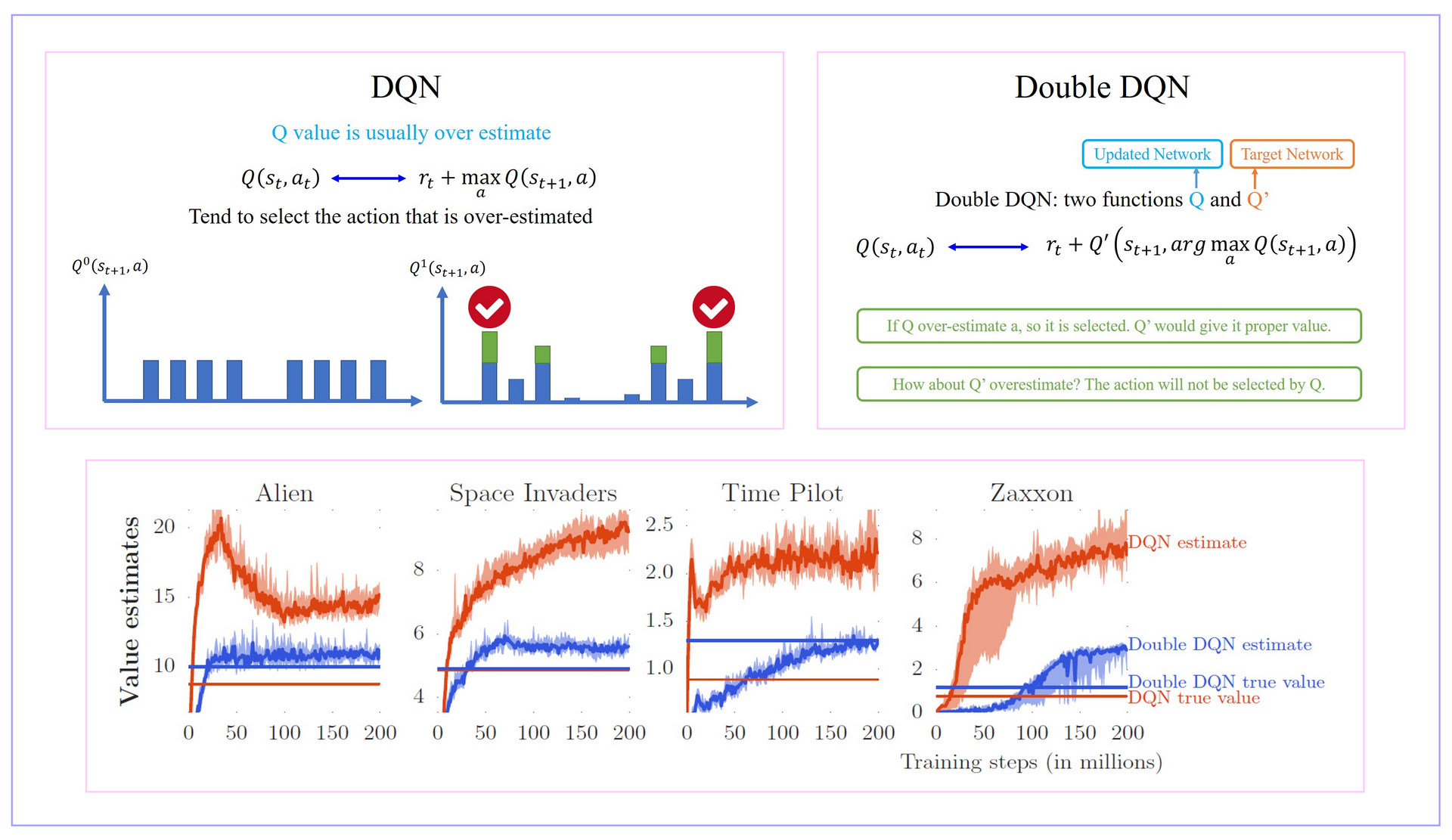4.2 Dueling DQN

Dueling DQN相比较DQN唯一优化的地方就是：修改Network的架构，可以更有效率地使用data来更新Network。

现在我们回到Qtable，虽然不精确，但Qtabel能够直观地把原理呈现出来。我们先改变Q值表的记录方式，原来我们会直接预估Q值表的数据，现在我们需要预估两个值：V值和A值。S值可以看成是该state下的Q值的平均数。A值是有所限制的，A值的平均数为0，V值与A值的和，就是原来的Q值。搞这么复杂有什么好处呢？我们仍然可以用理解Qlearning的方式，把图像可视化出来理解。

我们之前说过，DQN的Q网络，可以理解用一个曲线去拟合Qtable的Q值。现在我们取一个截面，表示当我们取某个V下，各个动作的Q值。

在普通DQN，当我们需要更新某个动作的Q值，我们会直接更新Q网络，令这个动作的Q值提升。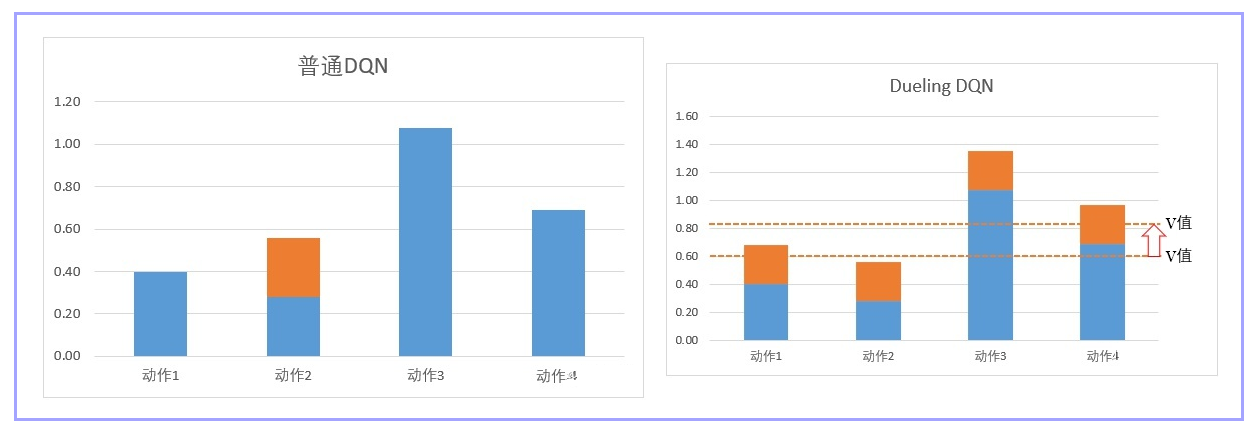如上图大家看到，一般DQN在提升某个状态下的S值时，只会提升某个动作。

但是在Dueling DQN中： 在网络更新的时候，由于有A值之和必须为0的限制，所以网络会优先更新V值。V值是Q值的平均数，平均数的调整相当于一次性V下的所有Q值都更新一遍。如上图，橙色虚线是平均值，也就是V值。所以网络在更新的时候，不但更新某个动作的Q值，而是把这个状态下，所有动作的Q值都调整一次。在图上就相当于直接提着橙色虚线调整。这样，我们就可以在更少的次数让更多的值进行更新。有同学可能会担心，这样调整最后的数值是对的吗？放心，在DuelingDQN，我们只是优先调整S值。但最终我们的target目标是没有变的，所以我们最后更新出来也是对的。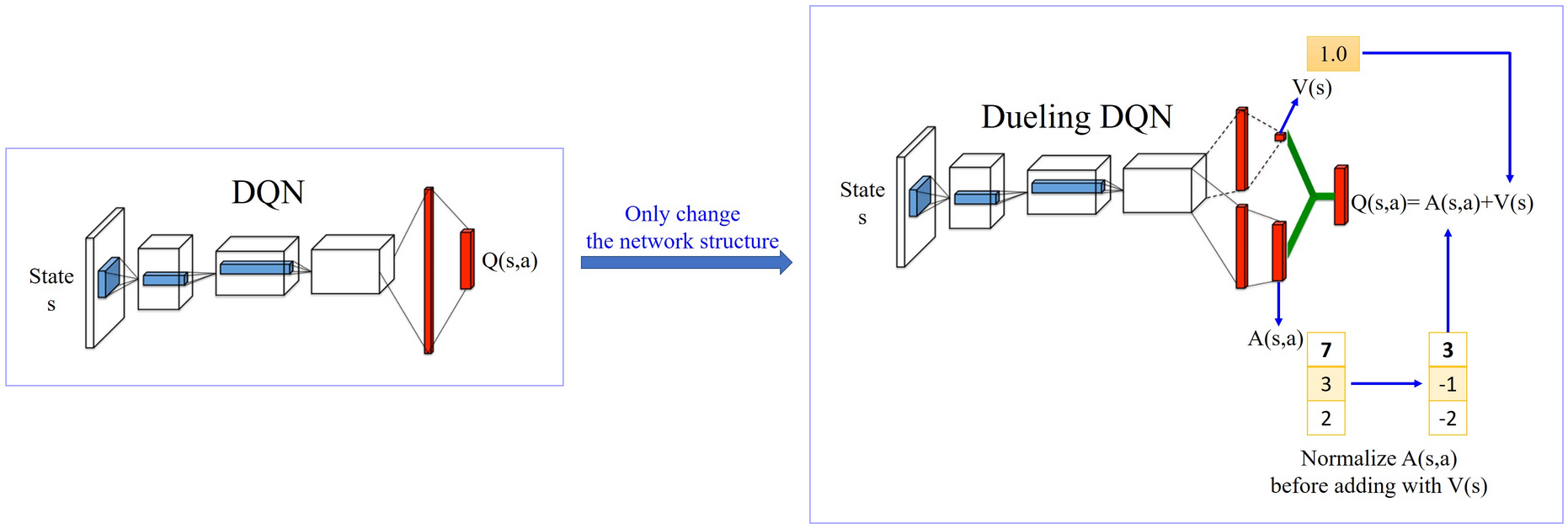DeulingDQN在实现的过程中，有可能说，反正 machine 就学到说我们也不要管什么 V 了，V 就永远都是 0。然后反正 A 就等于 Q，那你就没有得到任何 Dueling DQN 可以带给你的好处，就变成跟原来的 DQN 一模一样。所以为了避免这个问题，实际上你会对 A设定一些 constrain，你要给 A 一些 constrain，让 update A 其实比较麻烦，让 network 倾向于 会想要去用 V 来解问题。

我们可以把dueling DQN分为三部分。

第一部分：和普通DQN一样，用来处理和学习数据。
第二部分：计算 V 的值，就是让网络预估的平均值。
第三部分：计算A的值，和计算 V 的值一样，我们都是从h2层输入到该层。然后我们对A的值进行归一化处理，也就是增加“A值的平均值为0”的限制/Constrain。归一化的处理步骤是 network 的一部分，它没有参数，它就是一个 normalization 的 operation，那它可以放到 network 里面跟 network 的其他部分 jointly trained。这样 A 就会有比较大的 constrain，这样 network 就会给它一些 benefit，倾向于去 update V 的值。

DeulingDQN的实现很简单，只需要修改Q网络的网络架构就可以了。而且可以和其他DQN的技巧，例如经验回放，固定网络，双网络计算目标等可以共用。

参考资料：
深度强化学习——DQN
Playing Atari with Deep Reinforcement Learning
Human-level control through deep reinforcement learning
强化学习（十）Double DQN (DDQN)
强化学习（十）Double DQN (DDQN)
[番外篇]DuelingDQN为何那么强？(附代码及代码分析)


展开全文人工智能 强化学习
• 前言2013年DeepMind 在NIPS上发表Playing Atari with Deep Reinforcement Learning 一文，提出了DQN（Deep Q Network）算法，实现端到端学习玩Atari游戏，即只有像素输入，看着屏幕玩游戏。Deep Mind就凭借这个应用...
前言

2013年DeepMind 在NIPS上发表Playing Atari with Deep Reinforcement Learning 一文，提出了DQN（Deep Q Network）算法，实现端到端学习玩Atari游戏，即只有像素输入，看着屏幕玩游戏。Deep Mind就凭借这个应用以6亿美元被Google收购。由于DQN的开源，在github上涌现了大量各种版本的DQN程序。但大多是复现Atari的游戏，代码量很大，也不好理解。

Flappy Bird是个极其简单又困难的游戏，风靡一时。在很早之前，就有人使用Q-Learning 算法来实现完Flappy Bird。http://sarvagyavaish.github.io/FlappyBirdRL/
但是这个的实现是通过获取小鸟的具体位置信息来实现的。

能否使用DQN来实现通过屏幕学习玩Flappy Bird是一个有意思的挑战。（话说本人和朋友在去年年底也考虑了这个idea，但当时由于不知道如何截取游戏屏幕只能使用具体位置来学习，不过其实也成功了）

最近，github上有人放出使用DQN玩Flappy Bird的代码，https://github.com/yenchenlin1994/DeepLearningFlappyBird【1】
该repo通过结合之前的repo成功实现了这个想法。这个repo对整个实现过程进行了较详细的分析，但是由于其DQN算法的代码基本采用别人的repo，代码较为混乱，不易理解。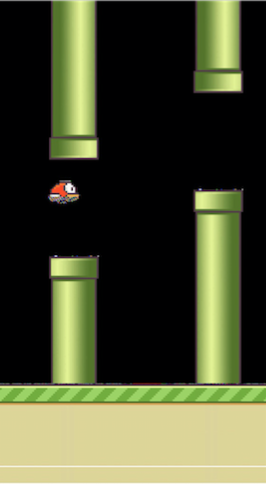为此，本人改写了一个版本https://github.com/songrotek/DRL-FlappyBird

对DQN代码进行了重新改写。本质上对其做了类的封装，从而使代码更具通用性。可以方便移植到其他应用。

当然，本文的目的是借Flappy Bird DQN这个代码来详细分析一下DQN算法极其使用。

DQN 伪代码

这个是NIPS13版本的伪代码：

Initialize replay memory D to size N
Initialize action-value function Q with random weights
for episode = 1, M do
Initialize state s_1
for t = 1, T do
With probability ϵ select random action a_t
otherwise select a_t=max_a  Q($s_t$,a; $θ_i$)
Execute action a_t in emulator and observe r_t and s_(t+1)
Store transition (s_t,a_t,r_t,s_(t+1)) in D
Sample a minibatch of transitions (s_j,a_j,r_j,s_(j+1)) from D
Set y_j:=
r_j for terminal s_(j+1)
r_j+γ*max_(a^' )  Q(s_(j+1),a'; θ_i) for non-terminal s_(j+1)
Perform a gradient step on (y_j-Q(s_j,a_j; θ_i))^2 with respect to θ
end for
end for

基本的分析详见Paper Reading 1 - Playing Atari with Deep Reinforcement Learning
基础知识详见Deep Reinforcement Learning 基础知识（DQN方面）

本文主要从代码实现的角度来分析如何编写Flappy Bird DQN的代码

编写FlappyBirdDQN.py

首先，FlappyBird的游戏已经编写好，是现成的。提供了很简单的接口：

nextObservation,reward,terminal = game.frame_step(action)

即输入动作，输出执行完动作的屏幕截图，得到的反馈reward，以及游戏是否结束。

那么，现在先把DQN想象为一个大脑，这里我们也用BrainDQN类来表示，这个类只需获取感知信息也就是上面说的观察（截图），反馈以及是否结束，然后输出动作即可。

完美的代码封装应该是这样。具体DQN里面如何存储。如何训练是外部不关心的。
因此，我们的FlappyBirdDQN代码只有如下这么短：

# -------------------------
# Project: Deep Q-Learning on Flappy Bird
# Author: Flood Sung
# Date: 2016.3.21
# -------------------------

import cv2
import sys
sys.path.append("game/")
import wrapped_flappy_bird as game
from BrainDQN import BrainDQN
import numpy as np

# preprocess raw image to 80*80 gray image
def preprocess(observation):
observation = cv2.cvtColor(cv2.resize(observation, (80, 80)), cv2.COLOR_BGR2GRAY)
ret, observation = cv2.threshold(observation,1,255,cv2.THRESH_BINARY)
return np.reshape(observation,(80,80,1))

def playFlappyBird():
# Step 1: init BrainDQN
brain = BrainDQN()
# Step 2: init Flappy Bird Game
flappyBird = game.GameState()
# Step 3: play game
# Step 3.1: obtain init state
action0 = np.array([1,0])  # do nothing
observation0, reward0, terminal = flappyBird.frame_step(action0)
observation0 = cv2.cvtColor(cv2.resize(observation0, (80, 80)), cv2.COLOR_BGR2GRAY)
ret, observation0 = cv2.threshold(observation0,1,255,cv2.THRESH_BINARY)
brain.setInitState(observation0)

# Step 3.2: run the game
while 1!= 0:
action = brain.getAction()
nextObservation,reward,terminal = flappyBird.frame_step(action)
nextObservation = preprocess(nextObservation)
brain.setPerception(nextObservation,action,reward,terminal)

def main():
playFlappyBird()

if __name__ == '__main__':
main()

核心部分就在while循环里面，由于要讲图像转换为80x80的灰度图，因此，加了一个preprocess预处理函数。

这里，显然只有有游戏引擎，换一个游戏是一样的写法，非常方便。

接下来就是编写BrainDQN.py 我们的游戏大脑

编写BrainDQN

基本架构：

class BrainDQN:
def __init__(self):
# init replay memory
self.replayMemory = deque()
# init Q network
self.createQNetwork()
def createQNetwork(self):

def trainQNetwork(self):

def setPerception(self,nextObservation,action,reward,terminal):
def getAction(self):
def setInitState(self,observation):

基本的架构也就只需要上面这几个函数，其他的都是多余了，接下来就是编写每一部分的代码。

CNN代码

也就是createQNetwork部分，这里采用如下图的结构（转自【1】）：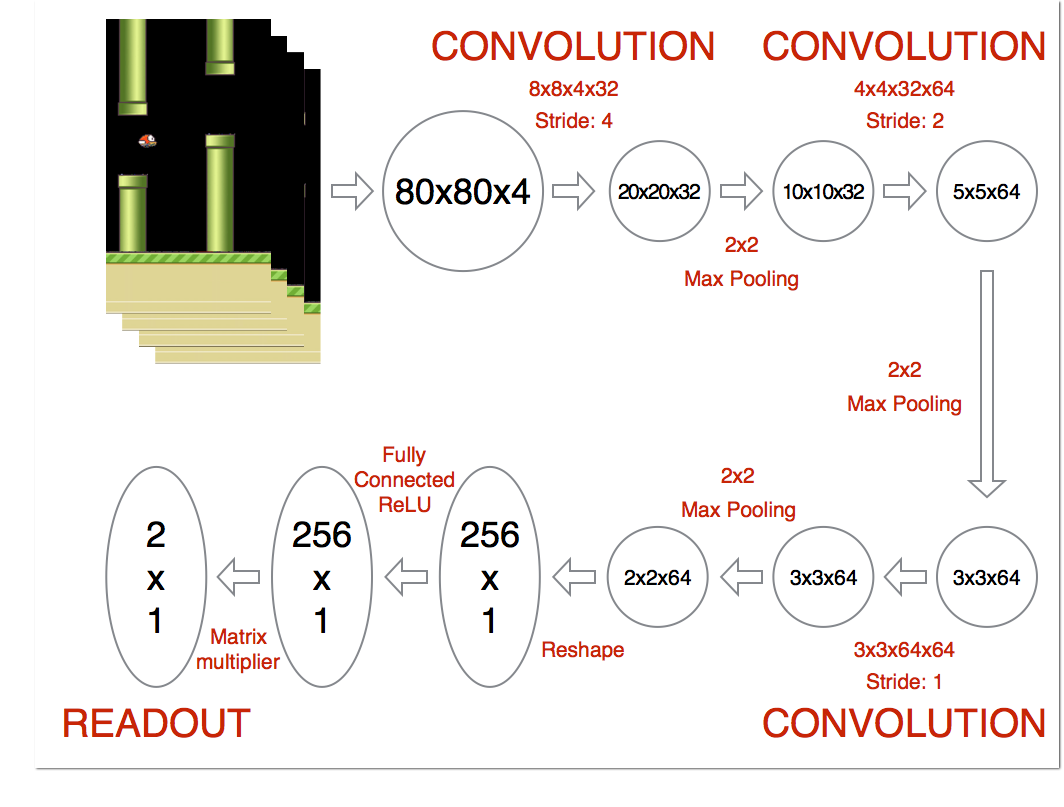这里就不讲解整个流程了。主要是针对具体的输入类型和输出设计卷积和全连接层。

代码如下：

    def createQNetwork(self):
# network weights
W_conv1 = self.weight_variable([8,8,4,32])
b_conv1 = self.bias_variable()

W_conv2 = self.weight_variable([4,4,32,64])
b_conv2 = self.bias_variable()

W_conv3 = self.weight_variable([3,3,64,64])
b_conv3 = self.bias_variable()

W_fc1 = self.weight_variable([1600,512])
b_fc1 = self.bias_variable()

W_fc2 = self.weight_variable([512,self.ACTION])
b_fc2 = self.bias_variable([self.ACTION])

# input layer

self.stateInput = tf.placeholder("float",[None,80,80,4])

# hidden layers
h_conv1 = tf.nn.relu(self.conv2d(self.stateInput,W_conv1,4) + b_conv1)
h_pool1 = self.max_pool_2x2(h_conv1)

h_conv2 = tf.nn.relu(self.conv2d(h_pool1,W_conv2,2) + b_conv2)

h_conv3 = tf.nn.relu(self.conv2d(h_conv2,W_conv3,1) + b_conv3)

h_conv3_flat = tf.reshape(h_conv3,[-1,1600])
h_fc1 = tf.nn.relu(tf.matmul(h_conv3_flat,W_fc1) + b_fc1)

# Q Value layer
self.QValue = tf.matmul(h_fc1,W_fc2) + b_fc2

self.actionInput = tf.placeholder("float",[None,self.ACTION])
self.yInput = tf.placeholder("float", [None])
Q_action = tf.reduce_sum(tf.mul(self.QValue, self.actionInput), reduction_indices = 1)
self.cost = tf.reduce_mean(tf.square(self.yInput - Q_action))
self.trainStep = tf.train.AdamOptimizer(1e-6).minimize(self.cost)

记住输出是Q值，关键要计算出cost，里面关键是计算Q_action的值，即该state和action下的Q值。由于actionInput是one hot vector的形式，因此tf.mul(self.QValue, self.actionInput)正好就是该action下的Q值。

training 部分。

这部分是代码的关键部分，主要是要计算y值，也就是target Q值。

    def trainQNetwork(self):
# Step 1: obtain random minibatch from replay memory
minibatch = random.sample(self.replayMemory,self.BATCH_SIZE)
state_batch = [data for data in minibatch]
action_batch = [data for data in minibatch]
reward_batch = [data for data in minibatch]
nextState_batch = [data for data in minibatch]

# Step 2: calculate y
y_batch = []
QValue_batch = self.QValue.eval(feed_dict={self.stateInput:nextState_batch})
for i in range(0,self.BATCH_SIZE):
terminal = minibatch[i]
if terminal:
y_batch.append(reward_batch[i])
else:
y_batch.append(reward_batch[i] + GAMMA * np.max(QValue_batch[i]))

self.trainStep.run(feed_dict={
self.yInput : y_batch,
self.actionInput : action_batch,
self.stateInput : state_batch
})

其他部分

其他部分就比较容易了，这里直接贴出完整的代码：

# -----------------------------
# File: Deep Q-Learning Algorithm
# Author: Flood Sung
# Date: 2016.3.21
# -----------------------------

import tensorflow as tf
import numpy as np
import random
from collections import deque

class BrainDQN:

# Hyper Parameters:
ACTION = 2
FRAME_PER_ACTION = 1
GAMMA = 0.99 # decay rate of past observations
OBSERVE = 100000. # timesteps to observe before training
EXPLORE = 150000. # frames over which to anneal epsilon
FINAL_EPSILON = 0.0 # final value of epsilon
INITIAL_EPSILON = 0.0 # starting value of epsilon
REPLAY_MEMORY = 50000 # number of previous transitions to remember
BATCH_SIZE = 32 # size of minibatch

def __init__(self):
# init replay memory
self.replayMemory = deque()
# init Q network
self.createQNetwork()
# init some parameters
self.timeStep = 0
self.epsilon = self.INITIAL_EPSILON

def createQNetwork(self):
# network weights
W_conv1 = self.weight_variable([8,8,4,32])
b_conv1 = self.bias_variable()

W_conv2 = self.weight_variable([4,4,32,64])
b_conv2 = self.bias_variable()

W_conv3 = self.weight_variable([3,3,64,64])
b_conv3 = self.bias_variable()

W_fc1 = self.weight_variable([1600,512])
b_fc1 = self.bias_variable()

W_fc2 = self.weight_variable([512,self.ACTION])
b_fc2 = self.bias_variable([self.ACTION])

# input layer

self.stateInput = tf.placeholder("float",[None,80,80,4])

# hidden layers
h_conv1 = tf.nn.relu(self.conv2d(self.stateInput,W_conv1,4) + b_conv1)
h_pool1 = self.max_pool_2x2(h_conv1)

h_conv2 = tf.nn.relu(self.conv2d(h_pool1,W_conv2,2) + b_conv2)

h_conv3 = tf.nn.relu(self.conv2d(h_conv2,W_conv3,1) + b_conv3)

h_conv3_flat = tf.reshape(h_conv3,[-1,1600])
h_fc1 = tf.nn.relu(tf.matmul(h_conv3_flat,W_fc1) + b_fc1)

# Q Value layer
self.QValue = tf.matmul(h_fc1,W_fc2) + b_fc2

self.actionInput = tf.placeholder("float",[None,self.ACTION])
self.yInput = tf.placeholder("float", [None])
Q_action = tf.reduce_sum(tf.mul(self.QValue, self.actionInput), reduction_indices = 1)
self.cost = tf.reduce_mean(tf.square(self.yInput - Q_action))
self.trainStep = tf.train.AdamOptimizer(1e-6).minimize(self.cost)

# saving and loading networks
saver = tf.train.Saver()
self.session = tf.InteractiveSession()
self.session.run(tf.initialize_all_variables())
checkpoint = tf.train.get_checkpoint_state("saved_networks")
if checkpoint and checkpoint.model_checkpoint_path:
saver.restore(self.session, checkpoint.model_checkpoint_path)
print "Successfully loaded:", checkpoint.model_checkpoint_path
else:
print "Could not find old network weights"

def trainQNetwork(self):
# Step 1: obtain random minibatch from replay memory
minibatch = random.sample(self.replayMemory,self.BATCH_SIZE)
state_batch = [data for data in minibatch]
action_batch = [data for data in minibatch]
reward_batch = [data for data in minibatch]
nextState_batch = [data for data in minibatch]

# Step 2: calculate y
y_batch = []
QValue_batch = self.QValue.eval(feed_dict={self.stateInput:nextState_batch})
for i in range(0,self.BATCH_SIZE):
terminal = minibatch[i]
if terminal:
y_batch.append(reward_batch[i])
else:
y_batch.append(reward_batch[i] + GAMMA * np.max(QValue_batch[i]))

self.trainStep.run(feed_dict={
self.yInput : y_batch,
self.actionInput : action_batch,
self.stateInput : state_batch
})

# save network every 100000 iteration
if self.timeStep % 10000 == 0:
saver.save(self.session, 'saved_networks/' + 'network' + '-dqn', global_step = self.timeStep)

def setPerception(self,nextObservation,action,reward,terminal):
newState = np.append(nextObservation,self.currentState[:,:,1:],axis = 2)
self.replayMemory.append((self.currentState,action,reward,newState,terminal))
if len(self.replayMemory) > self.REPLAY_MEMORY:
self.replayMemory.popleft()
if self.timeStep > self.OBSERVE:
# Train the network
self.trainQNetwork()

self.currentState = newState
self.timeStep += 1

def getAction(self):
QValue = self.QValue.eval(feed_dict= {self.stateInput:[self.currentState]})
action = np.zeros(self.ACTION)
action_index = 0
if self.timeStep % self.FRAME_PER_ACTION == 0:
if random.random() <= self.epsilon:
action_index = random.randrange(self.ACTION)
action[action_index] = 1
else:
action_index = np.argmax(QValue)
action[action_index] = 1
else:
action = 1 # do nothing

# change episilon
if self.epsilon > self.FINAL_EPSILON and self.timeStep > self.OBSERVE:
self.epsilon -= (self.INITIAL_EPSILON - self.FINAL_EPSILON)/self.EXPLORE

return action

def setInitState(self,observation):
self.currentState = np.stack((observation, observation, observation, observation), axis = 2)

def weight_variable(self,shape):
initial = tf.truncated_normal(shape, stddev = 0.01)
return tf.Variable(initial)

def bias_variable(self,shape):
initial = tf.constant(0.01, shape = shape)
return tf.Variable(initial)

def conv2d(self,x, W, stride):
return tf.nn.conv2d(x, W, strides = [1, stride, stride, 1], padding = "SAME")

def max_pool_2x2(self,x):
return tf.nn.max_pool(x, ksize = [1, 2, 2, 1], strides = [1, 2, 2, 1], padding = "SAME")



一共也只有160代码。
如果这个任务不使用深度学习，而是人工的从图像中找到小鸟，然后计算小鸟的轨迹，然后计算出应该怎么按键，那么代码没有好几千行是不可能的。深度学习大大减少了代码工作。

小结

本文从代码角度对于DQN做了一定的分析，对于DQN的应用，大家可以在此基础上做各种尝试。
展开全文深度增强学习 DQN
• DQN其实是深度学习和强化学习知识的结合，也就是用Deep Networks框架来近似逼近强化学习中的Q value。其中，使用的Deep Networks有两种框架，分别如下图所示： 框架1 框架1的输入是State和Action，State可以是一个...
转载自：https://www.jianshu.com/p/e6f5e9589285
概述
DQN其实是深度学习和强化学习知识的结合，也就是用Deep Networks框架来近似逼近强化学习中的Q value。其中，使用的Deep Networks有两种框架，分别如下图所示：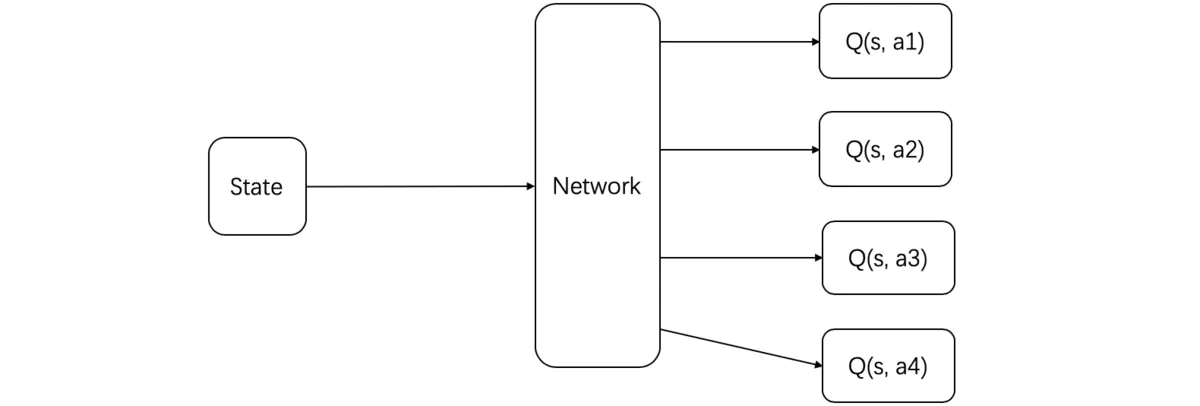框架1
框架1的输入是State和Action，State可以是一个游戏画面，Action可以是向下走，开火等，通过Network输出的是在State的情况下采取Action的Q value。框架2
框架2的输入是当前的State，通过Network输出的是在这个State的情况下，采取不同的Action所预测的Q value值，DQN paper中所采取的框架是框架2。
DQN是由两个Network组成，一个是Evaluation Network就是我们用来预测不同action的Q value值，另一个则是Target Network，是用来模拟真实的Q value值。DQN的loss就是一个L2 regression的loss，其公式如下：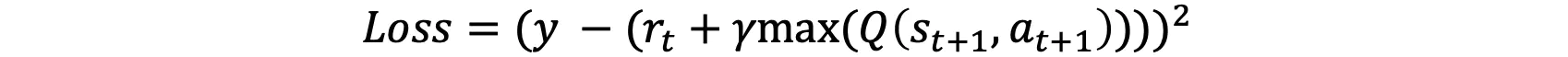loss function
y就是Evaluation Network要预测的Q(st, at)的值，其中st和at是已知的实验数据。剩下的减去的那一部分则是Target Network逼近的真实的Q(st, at)的值，rt，st+1是已知的，因此我们将st+1代入到Target Network中求最大的Q(st+1, at+1)的值即可。
=================================================================================
转载自： 莫烦Python   DQN
代码主结构
定义完上次的神经网络部分以后, 这次我们来定义其他部分. 包括:
class DeepQNetwork:
# 上次的内容
def _build_net(self):

# 这次的内容:
# 初始值
def __init__(self):

# 存储记忆
def store_transition(self, s, a, r, s_):

# 选行为
def choose_action(self, observation):

# 学习
def learn(self):

# 看看学习效果 (可选)
def plot_cost(self):

初始值
class DeepQNetwork:
def __init__(
self,
n_actions,
n_features,
learning_rate=0.01,
reward_decay=0.9,
e_greedy=0.9,
replace_target_iter=300,
memory_size=500,
batch_size=32,
e_greedy_increment=None,
output_graph=False,
):
self.n_actions = n_actions
self.n_features = n_features
self.lr = learning_rate
self.gamma = reward_decay
self.epsilon_max = e_greedy     # epsilon 的最大值
self.replace_target_iter = replace_target_iter  # 更换 target_net 的步数
self.memory_size = memory_size  # 记忆上限
self.batch_size = batch_size    # 每次更新时从 memory 里面取多少记忆出来
self.epsilon_increment = e_greedy_increment # epsilon 的增量
self.epsilon = 0 if e_greedy_increment is not None else self.epsilon_max # 是否开启探索模式, 并逐步减少探索次数

# 记录学习次数 (用于判断是否更换 target_net 参数)
self.learn_step_counter = 0

# 初始化全 0 记忆 [s, a, r, s_]
self.memory = np.zeros((self.memory_size, n_features*2+2)) # 和视频中不同, 因为 pandas 运算比较慢, 这里改为直接用 numpy

# 创建 [target_net, evaluate_net]
self._build_net()

# 替换 target net 的参数
t_params = tf.get_collection('target_net_params')  # 提取 target_net 的参数
e_params = tf.get_collection('eval_net_params')   # 提取  eval_net 的参数
self.replace_target_op = [tf.assign(t, e) for t, e in zip(t_params, e_params)] # 更新 target_net 参数

self.sess = tf.Session()

# 输出 tensorboard 文件
if output_graph:
# \$ tensorboard --logdir=logs
tf.summary.FileWriter("logs/", self.sess.graph)

self.sess.run(tf.global_variables_initializer())
self.cost_his = []  # 记录所有 cost 变化, 用于最后 plot 出来观看

创建网络
    def _build_net(self):
# -----------------------all inputs------------------------
self.s = tf.placeholder(tf.float32, [None, self.n_features], name='s')  # inout State
self.s_ = tf.placeholder(tf.float32, [None, self.n_features], name='s_')  # input next State
self.r = tf.placeholder(tf.float32, [None, ], name='r')  # input Reward
self.a = tf.placeholder(tf.int32, [None, ], name='a')  # inout Action

w_initializer, b_initializer = tf.random_normal_initializer(0., 0.3), tf.constant_initializer(0.1)

# -------------------------build evaluate net-----------------
with tf.variable_scope('eval_net'):
e1 = tf.layers.dense(self.s, 20, tf.nn.relu, kernel_initializer=w_initializer,
bias_initializer=b_initializer, name='e1')
self.q_eval = tf.layers.dense(e1, self.n_actions, kernel_initializer=w_initializer,
bias_initializer=b_initializer, name='q')

# ---------------------------build target net--------------------
with tf.variable_scope('target_net'):
t1 = tf.layers.dense(self.s_, 20, tf.nn.relu, kernel_initializer=w_initializer,
bias_initializer=b_initializer, name='t1')

self.q_next = tf.layers.dense(t1, self.n_actions, kernel_initializer=w_initializer,
bias_initializer=b_initializer, name='t2')

with tf.variable_scope('q_target'):
q_target = self.r + self.gamma * tf.reduce_max(self.q_next, axis=1, name='Qmax_s_')  # shape=(None, )
self.q_target = tf.stop_gradient(q_target)
with tf.variable_scope('q_eval'):
a_indices = tf.stack([tf.range(tf.shape(self.a), dtype=tf.int32), self.a], axis=1)
self.q_eval_wrt_a = tf.gather_nd(params=self.q_eval, indices=a_indices)  # shape=(None, )
with tf.variable_scope('loss'):
self.loss = tf.reduce_mean(tf.squared_difference(self.q_target, self.q_eval_wrt_a, name='TD_error'))
with tf.variable_scope('train'):
self._train_op = tf.train.RMSPropOptimizer(self.lr).minimize(self.loss)

存储记忆
DQN 的精髓部分之一: 记录下所有经历过的步, 这些步可以进行反复的学习, 所以是一种 off-policy 方法, 你甚至可以自己玩, 然后记录下自己玩的经历, 让这个 DQN 学习你是如何通关的.
class DeepQNetwork:
def __init__(self):
...
def store_transition(self, s, a, r, s_):
if not hasattr(self, 'memory_counter'):
self.memory_counter = 0

# 记录一条 [s, a, r, s_] 记录
transition = np.hstack((s, [a, r], s_))

# 总 memory 大小是固定的, 如果超出总大小, 旧 memory 就被新 memory 替换
index = self.memory_counter % self.memory_size
self.memory[index, :] = transition # 替换过程

self.memory_counter += 1

选行为
和之前的 QLearningTable, SarsaTable 等一样, 都需要一个选行为的功能.
class DeepQNetwork:
def __init__(self):
...
def store_transition(self, s, a, r, s_):
...
def choose_action(self, observation):
# 统一 observation 的 shape (1, size_of_observation)
observation = observation[np.newaxis, :]

if np.random.uniform() < self.epsilon:
# 让 eval_net 神经网络生成所有 action 的值, 并选择值最大的 action
actions_value = self.sess.run(self.q_eval, feed_dict={self.s: observation})
action = np.argmax(actions_value)
else:
action = np.random.randint(0, self.n_actions)   # 随机选择
return action

学习
最重要的一步来了, 就是在 DeepQNetwork 中, 是如何学习, 更新参数的. 这里涉及了 target_net 和 eval_net 的交互使用.
class DeepQNetwork:
def __init__(self):
...
def store_transition(self, s, a, r, s_):
...
def choose_action(self, observation):
...
def _replace_target_params(self):
...
def learn(self):
# 检查是否替换 target_net 参数
if self.learn_step_counter % self.replace_target_iter == 0:
self.sess.run(self.replace_target_op)
print('\ntarget_params_replaced\n')

# 从 memory 中随机抽取 batch_size 这么多记忆
if self.memory_counter > self.memory_size:
sample_index = np.random.choice(self.memory_size, size=self.batch_size)
else:
sample_index = np.random.choice(self.memory_counter, size=self.batch_size)
batch_memory = self.memory[sample_index, :]

# 获取 q_next (target_net 产生了 q) 和 q_eval(eval_net 产生的 q)
q_next, q_eval = self.sess.run(
[self.q_next, self.q_eval],
feed_dict={
self.s_: batch_memory[:, -self.n_features:],
self.s: batch_memory[:, :self.n_features]
})

# 下面这几步十分重要. q_next, q_eval 包含所有 action 的值,
# 而我们需要的只是已经选择好的 action 的值, 其他的并不需要.
# 所以我们将其他的 action 值全变成 0, 将用到的 action 误差值 反向传递回去, 作为更新凭据.
# 这是我们最终要达到的样子, 比如 q_target - q_eval = [1, 0, 0] - [-1, 0, 0] = [2, 0, 0]
# q_eval = [-1, 0, 0] 表示这一个记忆中有我选用过 action 0, 而 action 0 带来的 Q(s, a0) = -1, 所以其他的 Q(s, a1) = Q(s, a2) = 0.
# q_target = [1, 0, 0] 表示这个记忆中的 r+gamma*maxQ(s_) = 1, 而且不管在 s_ 上我们取了哪个 action,
# 我们都需要对应上 q_eval 中的 action 位置, 所以就将 1 放在了 action 0 的位置.

# 下面也是为了达到上面说的目的, 不过为了更方面让程序运算, 达到目的的过程有点不同.
# 是将 q_eval 全部赋值给 q_target, 这时 q_target-q_eval 全为 0,
# 不过 我们再根据 batch_memory 当中的 action 这个 column 来给 q_target 中的对应的 memory-action 位置来修改赋值.
# 使新的赋值为 reward + gamma * maxQ(s_), 这样 q_target-q_eval 就可以变成我们所需的样子.
# 具体在下面还有一个举例说明.

q_target = q_eval.copy()
batch_index = np.arange(self.batch_size, dtype=np.int32)
eval_act_index = batch_memory[:, self.n_features].astype(int)
reward = batch_memory[:, self.n_features + 1]

q_target[batch_index, eval_act_index] = reward + self.gamma * np.max(q_next, axis=1)

"""
假如在这个 batch 中, 我们有2个提取的记忆, 根据每个记忆可以生产3个 action 的值:
q_eval =
[[1, 2, 3],
[4, 5, 6]]

q_target = q_eval =
[[1, 2, 3],
[4, 5, 6]]

然后根据 memory 当中的具体 action 位置来修改 q_target 对应 action 上的值:
比如在:
记忆 0 的 q_target 计算值是 -1, 而且我用了 action 0;
记忆 1 的 q_target 计算值是 -2, 而且我用了 action 2:
q_target =
[[-1, 2, 3],
[4, 5, -2]]

所以 (q_target - q_eval) 就变成了:
[[(-1)-(1), 0, 0],
[0, 0, (-2)-(6)]]

最后我们将这个 (q_target - q_eval) 当成误差, 反向传递会神经网络.
所有为 0 的 action 值是当时没有选择的 action, 之前有选择的 action 才有不为0的值.
我们只反向传递之前选择的 action 的值,
"""

# 训练 eval_net
_, self.cost = self.sess.run([self._train_op, self.loss],
feed_dict={self.s: batch_memory[:, :self.n_features],
self.q_target: q_target})
self.cost_his.append(self.cost) # 记录 cost 误差

# 逐渐增加 epsilon, 降低行为的随机性
self.epsilon = self.epsilon + self.epsilon_increment if self.epsilon < self.epsilon_max else self.epsilon_max
self.learn_step_counter += 1



展开全文深度学习 机器学习
• Deep Q Network 的简称叫 DQN, 是将 Q learning 的优势 和 Neural networks 结合了. 如果我们使用 tabular Q learning, 对于每一个 state, action 我们都需要存放在一张 q_table 的表中. 如果像显示生活中, 情况可就...强化学习
• Just like Keras, it works with either [Theano](http://deeplearning.net/software/theano/) or [TensorFlow](https://www.tensorflow.org/), which means that you can train your algorithm efficiently either...
• <div><p>I noticed that -1511 implemented a Deep Q-...<p>Could you provide official support for Deep Q-learning in the master branch?</p><p>该提问来源于开源项目：NervanaSystems/neon</p></div>
• Q-Learning epsilon greedy strategy ...Deep Reinforcement Learning: Guide to Deep Q-Learning 强化学习——从Q-Learning到DQN到底发生了什么？ Deep Q Learning之小白 价值函数的近似表示与Deep Q-Learning ...
Q-Learning

epsilon greedy strategy
freecodecamp
时序差分离线控制算法Q-Learning

Deep Q-Learning

Deep Reinforcement Learning: Guide to Deep Q-Learning
强化学习——从Q-Learning到DQN到底发生了什么？
Deep Q Learning之小白
价值函数的近似表示与Deep Q-Learning


展开全文强化学习
• Deep Learning 定义 深度学习(Deep Learing)由一组算法和技术构成，这些算法和技术试图发现数据的重要特征并对其高级抽象建模。深度学习的主要目标是通过对数据的自动学习来避免手动描述数据结构(如手写特征)。深度...神经网络 算法 python 机器学习 深度学习
• 月球兰德 使用pytorch中的Double Deep Q-Learning教AI安全着陆飞船。
• 深度强化学习最新经典论文 Exploring Deep Reinforcement Learning with Multi Q-Learning强化学习
• 使用sumo仿真的强化学习deep-qlearning 可用于本科机器学习入门学习
• DQ深度学习Deep Reinforcement Learning with Double Q-Learning.pdf深度学习
• 这篇博文前半部分介绍DNN、CNN熟悉这些的读者可以跳过，直接看后半部分的Deep Q Learning Part。 Generalization 为什么要使用网络来做近似。 希望能使用强化学习来处理自动驾驶汽车，Atari，消费者市场，医疗...DQN
• 深度强化学习之Deep Q Learning 本文主要讲解：Deep Q Network 下面我们简称为 DQN。DQN采用深度神经网络来表示Q函数，通常也称为Deep Q Learning 。 DQN是在论文《Playing Atari with Deep Reinforcement Learning...DQN 强化学习
• Q learning: 1 主要用在解是离散时 2 主要是利用值函数,即,直接由值函数来推策略 3 其核心在于bellman方程和代价函数 bellman的核心在于使用reward的时候要考虑到将来的情况,而不是只考虑现在的情况,否则的话,只...DQN 强化学习
• Deep Q Learning Generalization Deep Reinforcement Learning 使用深度神经网络来表示 价值函数 策略 模型 使用随机梯度下降(SGD)优化loss函数 Deep Q-Networks(DQNs) 使用带权重集w\textbf{w}w的Q-network...DQN Double DQN
• Deep Reinforcement Learning with Double Q-learning Google DeepMind Abstract 　主流的 Q-learning 算法过高的估计在特定条件下的动作值。实际上，之前是不知道是否这样的过高估计是 common的，是否对性...
• 零基础10分钟运行DQN图文教程 Playing Flappy Bird Using Deep Reinforcement Learning (Based on Deep Q Learning DQN)机器学习 DQN
• An introduction to Deep Q-Learning: let’s playDoom 在上一篇博文，我们学习了Q-learning这种用来产生Q-table的算法。但是我们可以发现，产生和更新Q-table的过程在大的状态空间环境下效率低。 在这篇文章中，...
• 深度Q强化学习 描述 强化学习可以用作训练代理在给定环境下玩游戏的技术。 Q学习是强化学习的一种简单类型，由此创建了一个“ Q表”，其中包含AI对每个游戏状态下代理可使用的每个动作的“质量”（因此称为名称）的...Python
• You will also see applications of DL for controlling robotics, exploring the DeepQ learning algorithm with Monte Carlo Tree search (used to beat humans in the game of Go), and modeling for financial ...深度学习
• 首先要明白一个前提，这里介绍的是没有使用神经网络的qlearning，而不是Deep QLearning。而且算法不使用梯度下降，而是迭代更新。 一、算法详解 1.1 几个概念 1.1.1 什么是critic？ critic：批评家，评论家。 在这里...强化学习 机器学习 神经网络 python
• In particular, we first show that the recent DQN algorithm, which combines Q-learning with a deep neural network, suffers from substantial overestimations in some games in the Atari 2600 domain.......